• 第一板斧：上下三角分块 第二板斧： 对角为0零的分块 第三板斧： 全分块 小招：A^2 - B^2 其他招式： 利用特征值计算行列式
第一板斧：上下三角分块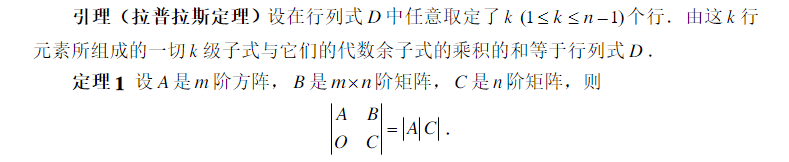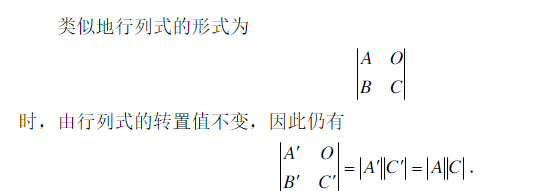第二板斧： 对角为0零的分块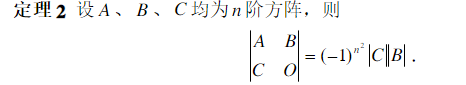第三板斧： 全分块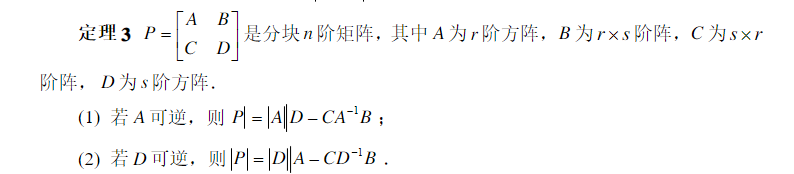小招：A^2 - B^2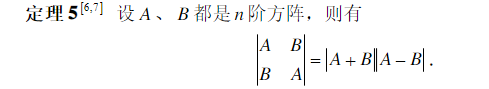其他招式： 利用特征值计算行列式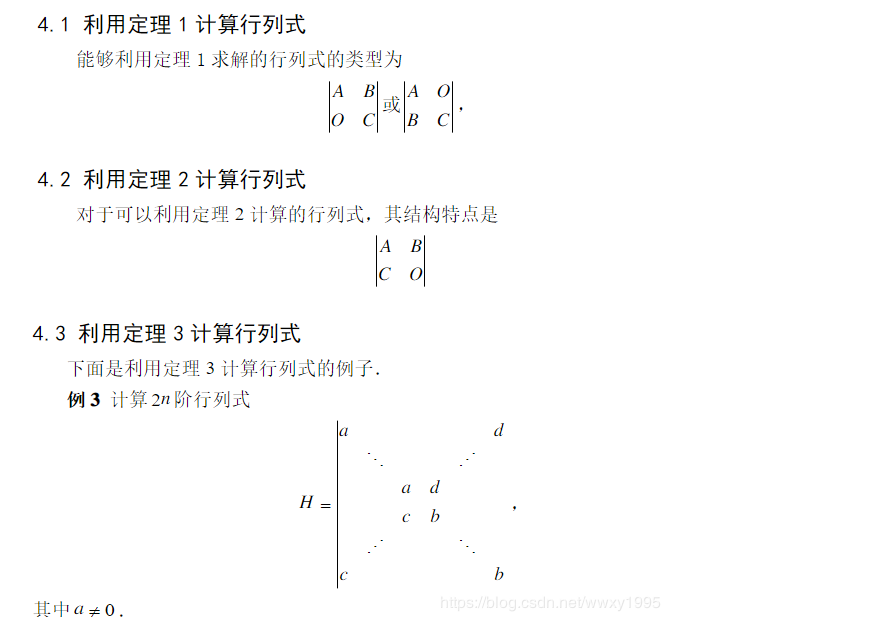展开全文• 一般的三阶矩阵求特征值其实是解析不了的，因为特征方程对应的是三次方程，对于一般的三次方程，是很难求解的。要想方程有三个整数根，并且能够不用完全暴力展开三阶行列式这样的矩阵实际是很特殊的。 1.某一行有...
一般的三阶矩阵求特征值其实是解析不了的，因为特征方程对应的是三次方程，对于一般的三次方程，是很难求解的。要想方程有三个整数根，并且能够不用完全暴力展开三阶行列式这样的矩阵实际是很特殊的。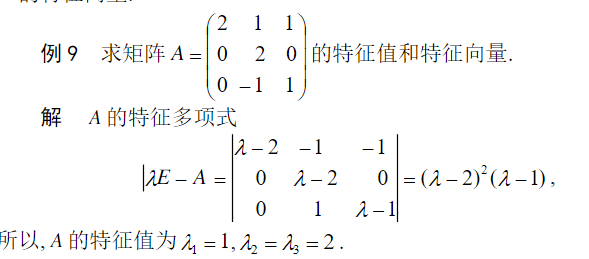1.某一行有两个0的情况是最好算的情况(分块上/下三角），考研中一般不会这么出，因为这样就能直接求出一个根，以及一个特征向量。可以拆分为一个稚为一的矩阵加上对角矩阵的矩阵。这样的矩阵也可以口算出来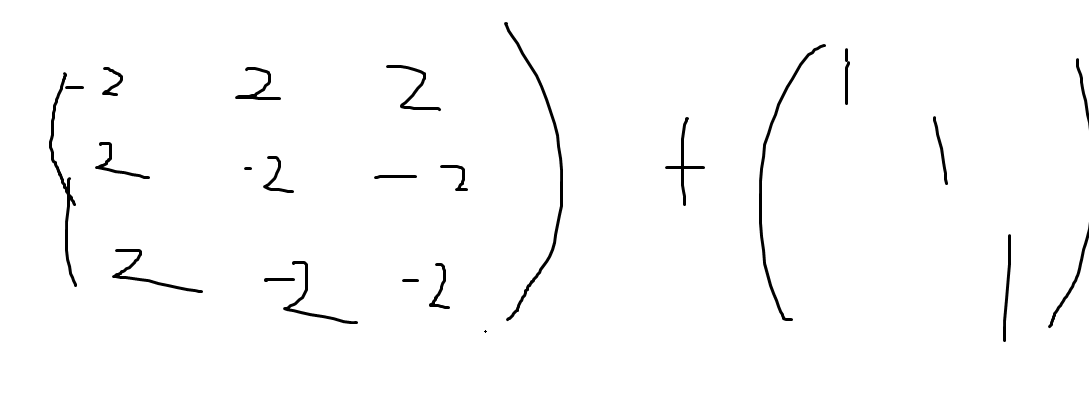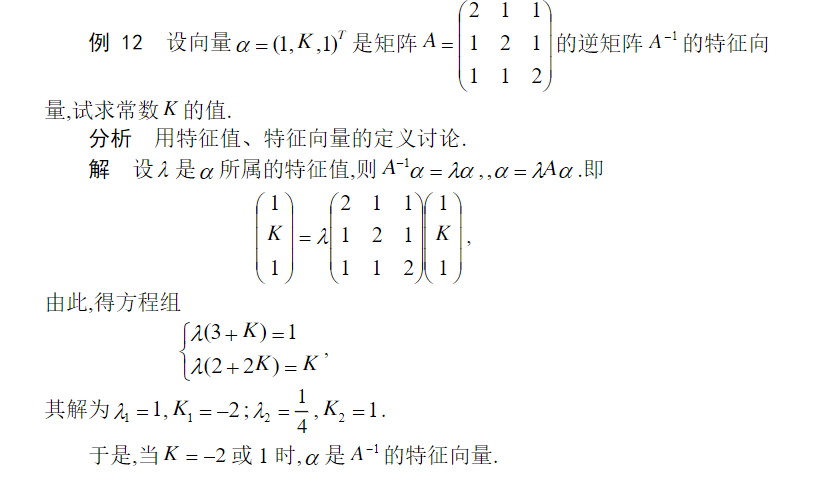展开全文• 文章目录一、行列式-计算方法与重要性质二、矩阵的秩，特征值与特征多项式 一、行列式-计算方法与重要性质 行列式定义\color{red}\textbf{行列式定义}行列式定义 行列式的定义依赖于逆序数与全排列， 行列式计算...
马上要开始一大波夏令营面试了，前不久thu叉院的一面问到了概率分布，没有准备好，用了一周左右的时间断断续续的复习了一下线性代数，后面再概率论吧，主要总结了一些基础知识，概念和性质。
文章目录一、行列式-计算方法与重要性质二、矩阵的秩，特征值与特征多项式三、逆，奇异，正交，伴随，实对称，正定矩阵四、向量组与线性相关（无关）五、线性方程组的解，与秩的关系六、二次型的基本内容和重要结论
一、行列式-计算方法与重要性质
$\color{red}\textbf{行列式定义}$
行列式的定义依赖于逆序数与全排列，需要注意的是，行列式只是方阵的概念。$\color{red}\textbf{行列式计算以及性质}$
行列式的计算除了直接用定义以外，可以使用如下性质进行计算的简化。

1、三角形行列式的值，等于对角线元素的乘积。计算时，一般需要多次运算来把行列式转换为上三角型或下三角型
2、交换行列式中的两行（列），行列式变号（交换）
3、行列式中某行（列）的公因子，可以提出放到行列式之外。（倍乘）（注：矩阵是全部元素都乘，都提取）
4、行列式的某行乘以a，加到另外一行，行列式不变，常用于消去某些元素。（倍加）
5、若行列式中，两行（列）完全一样，则行列式为0；可以推论，如果两行（列）成比例，行列式为0。
6、行列式展开：行列式的值，等于其中某一行（列）的每个元素与其代数余子式乘积的和；但若是另一行（列）的元素与本行（列）的代数余子式乘积求和，则其和为0

$\color{red}\textbf{行列式重要公式}$拉普拉斯展开式中，m，n分别是A,B矩阵的阶数。

$\color{red}\textbf{方阵的行列式}$

$|A^T|=|A|$
$|kA|=k^n|A|$
$|AB|=|A||B|$
$|A^*|=|A|^{n-1}$，A是n阶矩阵。
$|A|^{-1}=|A^{-1}|$
A的行列数是A所有特征值的乘积。

相关博文：

二、矩阵的秩，特征值与特征多项式
矩阵的特征值刻画矩阵的奇异性、反映矩阵所有对角元素的结构、刻画矩阵的正定性。

$\color{red}\textbf{秩}$
一个矩阵A的列秩是A的线性独立的纵列的极大数目。类似地，行秩是A的线性无关的横行的极大数目。即如果把矩阵看成一个个行向量或者列向量，秩就是这些行向量或者列向量的秩，也就是极大无关组中所含向量的个数。
如果A中，存在一个i阶子式不为0，且所有i+1阶子式对应的行列式值为0，那么r(A)=i（所谓的i阶子式即在矩阵中人去一个i*i的方阵）
求矩阵的秩时，除了利用定义法和上面的观察法，主要是通过性质，经过初等变换，矩阵秩不变。若A可逆，则r(AB)=r(BA)=r(B)

$\color{red}\textbf{特征值与特征向量}$物理意义：我们可以将矩阵看成是一个力的混合体，但需要注意的是，这个力的混合体中各个力是相互独立的！即特征向量之间线性无关，是无法做力的合成（这里只是假设其无法合成，有更好的解释以后会补充）的。其中力的个数为矩阵的秩，力的大小为特征值的大小，力的方向即为特征向量的方向。
详细解释见深度理解矩阵的奇异值，特征值
$\color{red}\textbf{特征多项式}$
A为n阶矩阵，若数λ和n维非0列向量x满足Ax=λx，那么数λ称为A的特征值，x称为A的对应于特征值λ的特征向量。式Ax=λx也可写成( A-λE)x=0，并且|λE-A|叫做A 的特征多项式。当特征多项式等于0的时候，称为A的特征方程，特征方程是一个齐次线性方程组，求解特征值的过程其实就是求解特征方程的解。$\color{red}\textbf{特征值相关的重要性质}$

设$A$是n阶矩阵，$\lambda_1,..,\lambda_n$是矩阵$A$的特征值，那么我们有如下两条性质$1:\sum\lambda_i=\sum a_{ii}，2:\prod\lambda_i=|A|$。
不同特征值对应的特征向量线性无关。
实对阵矩阵$A$的不同特征值$\lambda_i$所对应的特征向量$\alpha_i$必然正交。实对称矩阵A的特征值都是实数，特征向量都是实向量。
下三角矩阵，上三角矩阵，对角矩阵的特征值就是矩阵主对角线上的元素。

三、逆，奇异，正交，伴随，实对称，正定矩阵
$\color{red}\textbf{理解矩阵}$
一片很好的文章，在线性空间中，当你选定一组基之后，不仅可以用一个向量来描述空间中的任何一个对象，而且可以用矩阵来描述该空间中的任何一个运动（变换）。而使某个对象发生对应运动的方法，就是用代表那个运动的矩阵，乘以代表那个对象的向量。
简而言之，在线性空间中选定基之后，向量刻画对象，矩阵刻画对象的运动，用矩阵与向量的乘法施加运动。这就是线性代数中所说的坐标变换。
是的，矩阵的本质是运动的描述。如果以后有人问你矩阵是什么，那么你就可以响亮地告诉他，矩阵的本质是运动的描述。（chensh，说你呢！）

$\color{red}\textbf{矩阵的逆以及计算}$
设$A$是$n$阶矩阵，如果存在$n$阶矩阵$B$，使得$AB=BA=E(单位矩阵)$成立，那么称$A$为可逆矩阵或者非奇异矩阵。
求出逆矩阵的3种手算方法：

待定系数法：对矩阵$A$，直接设一个全为未知数的矩阵$B$，使得$AB=E$，解方程得到$B$的所有值。
初等变换法（初等行变化用的比较多），将矩阵$A$，增广为$A|E$的形式，通过初等变化将其变为$EA^{-1}$。

这三种方法百度百科讲的无比清楚

$\color{red}\textbf{奇异矩阵和非奇异矩阵}$
以下内容来自于这里
首先需要说明的值奇异矩阵和非奇异矩阵都是针对方阵而言的。奇异矩阵是线性代数的概念，就是对应的行列式等于0的矩阵。

非奇异矩阵的英文是nonsingular matrices，从对应的英文单词nonsingular上来讲，singular有一个含义是单数的，那么nonsingular是非单数，与非奇异矩阵的性质对上了，即有矩阵A，矩阵B，满足条件:AB=BA=I，I是一个单元矩阵，那么矩阵A和矩阵B均为非奇异矩阵。非奇异，即A不是单个的，是成对的。

奇异矩阵的判定方法：

行列式|A|是否等于0，若等于0，称矩阵A为奇异矩阵；

非奇异矩阵的判定方法：

一个矩阵非奇异当且仅当它的行列式不为零。
一个矩阵非奇异当且仅当它代表的线性变换是个自同构。
一个矩阵非奇异当且仅当它的秩为n。
(R(A)<n则行列式为0) 可逆矩阵就是非奇异矩阵，非奇异矩阵也是可逆矩阵。**

$\color{red}\textbf{正交矩阵及其性质}$
如果：$AA^T=E$（E为单位矩阵，$A^T$表示“矩阵A的转置矩阵”。）或$A^TA=E$，则$n$阶实矩阵$A$称为正交矩阵。
正交矩阵的性质：

1)$A^T$是正交矩阵
2)$A$的各行是单位向量且两两正交
3)$A$的各列是单位向量且两两正交
4)$|A|=1$或-1

$\color{red}\textbf{实对称矩阵}$

如果有n阶矩阵A，其矩阵的元素都为实数，且矩阵A的转置等于其本身（aij=aji）(i,j为元素的脚标），则称A为实对称矩阵。
主要性质：
1.实对称矩阵A的不同特征值对应的特征向量是正交的。
2.实对称矩阵A的特征值都是实数，特征向量都是实向量。
3.n阶实对称矩阵A必可相似对角化，且相似对角阵上的元素即为矩阵本身特征值。
4.若A具有k重特征值λ0　必有k个线性无关的特征向量，或者说秩r(λ0E-A)至多为n-k，其中E为单位矩阵。

$\color{red}\textbf{正定，半正定矩阵定义与重要性质}$

在线性代数里，正定矩阵 (positive definite matrix) 有时会简称为正定阵。在线性代数中，正定矩阵的性质类似复数中的正实数。与正定矩阵相对应的线性算子是对称正定双线性形式（复域中则对应埃尔米特正定双线性形式）。

（1）广义定义：设$M$是$n$阶方阵，如果对任何非零向量$z$，都有$\mathbf{z^TMz> 0}$，其中$z^T$ 表示$z$的转置，就称$M$为正定矩阵。
例如：B为n阶矩阵，E为单位矩阵，a为正实数。在a充分大时，aE+B为正定矩阵。（B必须为对称阵）
（2）狭义定义：一个$n$阶的实对称矩阵M是正定的的条件是当且仅当对于所有的非零实系数向量z，都有$z^TMz> 0$。其中$z^T$表示$z$的转置。

重要性质：
正定矩阵有以下性质   ：
（1）正定矩阵的行列式恒为正；
（2）实对称矩阵A正定当且仅当A与单位矩阵合同；
（3）若A是正定矩阵，则A的逆矩阵也是正定矩阵；
（4）两个正定矩阵的和是正定矩阵；
（5）正实数与正定矩阵的乘积是正定矩阵。

等价命题：
对于n阶实对称矩阵A，下列条件是等价的：
（1）A是正定矩阵；
（2）A的一切顺序主子式均为正；
（3）A的一切主子式均为正；
（4）A的特征值均为正；
（5）存在实可逆矩阵C，使A=C′C；
（6）存在秩为n的m×n实矩阵B，使A=B′B；
（7）存在主对角线元素全为正的实三角矩阵R，使A=R′R   。

判定方法：
根据正定矩阵的定义及性质，判别对称矩阵A的正定性有两种方法：
（1）求出A的所有特征值。若A的特征值均为正数，则A是正定的；若A的特征值均为负数，则A为负定的。
（2）计算A的各阶主子式。若A的各阶主子式均大于零，则A是正定的；若A的各阶主子式中，奇数阶主子式为负，偶数阶为正，则A为负定的。
四、向量组与线性相关（无关）
$\color{red}\textbf{线性无关的定义}$
在线性代数里，矢量空间的一组元素中，若没有矢量可用有限个其他矢量的线性组合所表示，则称为线性无关或线性独立 (linearly independent)，反之称为线性相关(linearly dependent)。
线性相关(Linear dependent)与线性无关(Linear independent)对于理解子空间的基，子空间的维数，以及矩阵的秩等等是重要的.
数学定义：
如果线性空间X中的向量组$\mathbf{x_1,x_2,...,x_j}$存在如下线性关系：
$\mathbf{k_1x_1+k_2x_2+,...,+k_jx_j=0, }$
其中$k_1,...,k_j$为不全为零的实数.则称$\mathbf{x_1,x_2,...,x_j}$线性相关.如果只有当$k_1,...,k_j$全为零时才满足上式,则称$\mathbf{x_1,x_2,...,x_j}$线性无关.

$\color{red}\textbf{向量组的秩}$
通俗的说，就是把这一组向量中的垃圾向量踢出后剩下的高品质向量的个数，假设这一组有5个向量，踢出两个垃圾，还剩3个。
那么这个向量组的秩就是3。那什么是垃圾向量呢？就是能被别人线性表示的向量。比如说向量α1能被α2和α3线性表示，也就是它的工作能被别人取代。那么α1就是垃圾向量！
正式定义：
一个向量组的极大线性无关组所包含的向量的个数，称为向量组的秩；若向量组的向量都是0向量，则规定其秩为0.向量组α1，α2，···，αs的秩记为R{α1，α2，···，αs}或rank{α1，α2，···，αs}。
极大线性无关组：

极大线性无关组（maximal linearly independent system）是线性空间的基对向量集的推广。设V是域P上的线性空间，S是V的子集。若S的一部分向量线性无关，但在这部分向量中，加上S的任一向量后都线性相关，则称这部分向量是S的一个极大线性无关组。V中子集的极大线性无关组不是惟一的，例如，V的基都是V的极大线性无关组。它们所含的向量个数（基数）相同。V的子集S的极大线性无关组所含向量的个数（基数），称为S的秩。只含零向量的子集的秩是零。V的任一子集都与它的极大线性无关组等价。特别地，当S等于V且V是有限维线性空间时，S的秩就是V的维数。–百度百科

五、线性方程组的解，与秩的关系
先给出两个写的很好的blog，1，2，然后结合他俩&书总结一下。
$\color{red}\textbf{线性方程组什么时候无解？多个解？唯一解?}$
$非齐次线性方程组$
非齐次线性方程组:化简后的有效方程组个数小于未知数个数，有多个解。
非齐次线性方程组:化简后的有效方程组个数等于未知数个数，有唯一解。
非齐次线性方程组:化简后的有效方程组出现(0=d)型式不兼容方程，则无解 。
下面从左到右依次是原方程，增广矩阵（非齐次线性方程组，就是方程组的等式右边不为0的方程组，系数加上方程等式右边的矩阵，叫做增广矩阵），以及化简后的增广矩阵，化简后的方程组。这样，x2可以通过x3来表示，x1也可以通过x3来表示，这样x3就叫做自由变量，x3可以取任意值。所以x1,x2,x3就有无穷多个解。即化简后的有效方程组个数，小于未知数个数。这样的方程组有无穷多个解

$齐次线性方程组$
齐次线性方程组，就是方程组的等式右边全部是0的方程组，只有系数矩阵，不需要增广矩阵，所以不会出现{0=d}形式的不相容方程。所以不会出现无解的情况，那么显然，齐次线性方程组的秩与其系数矩阵的秩肯定是相等（因为增广了一列0，不影响秩的，也就是说它肯定有解。这个也好理解，零向量肯定是他的解嘛。关键问题在于，它什么时候会有非零解。
对于Ax=0的齐次线性方程组，列出其系数矩阵（不需要增广矩阵），使用高斯消元法化简，化为阶梯形矩阵，化简后，判断有效方程组个数是否小于未知数个数，
如果有效方程组个数小于未知数个数，叫做有非零解（多个解）
如果等于，叫做只有零解（唯一解）

$\color{red}\textbf{通过矩阵的秩判断线性方程组的解}$
线性方程组什么时候无解，有多个解，唯一解？
对于非齐次线性方程组，用矩阵的秩r(A)来判断
对线性方程组进行初等变换（高斯消元法），化为最简型（阶梯形）矩阵，
考查系数矩阵$r(A)$，增广矩阵$r(A,b)$，以及方程组未知数个数$n$

如果系数矩阵的秩$r(A)$小于增广矩阵的秩$r(A,b)$，$r(A)，那么方程组无解$r(A)，那么方程组无解，即$b$不能由$A$的列向量线性表出；
如果系统矩阵的秩小于方程组未知数个数，$r(A)=r(A,b)，那么方程组有多个解$r(A)=r(A,b)，那么方程组有多个解。
如果系统矩阵的秩等于方程组未知数个数，$r(A)=r(A,b)=n$，那么方程组有唯一解$r(A)=r(A,b)=n$，那么方程组有唯一解。

对于齐次线性方程组，用行列式的值 detA来判断。

不存在无解的情况

$r(A)时，等价于$A$的列向量线性相关，那么方程的数目小于未知数的数目，一定有非零解。

$r(A)=n$，即$|A|≠0$，$A$满秩，则只有零解（只有唯一解）

设齐次方程组系数矩阵的秩$r(A)=r，则$Ax=0$的基础解系由$n-r(A)$个线性无关的解向量所构成。

$\color{red}\textbf{线性方程组的求解}$
写出系数矩阵 -> 行初等变换为行简化矩阵 -> 求基础解系 -> 写出通解
这个例子还不错，就是增广矩阵不断的进行初等变换，化为行最简矩阵（在阶梯形矩阵中，若非零行的第一个非零元素全是1，且非零行的第一个元素1所在列的其余元素全为零，就称该矩阵为行最简形矩阵。）然后每个方程中的第一个未知量通常称为主变量，其余的未知量称之为自由变量。对自由变量$x_1,...,x_k$依次取1，其余取0时求得的解向量即方程的一个解向量，有多少个自由变量，就能求出多少解向量。总结一下：对非齐次线性方程组而言：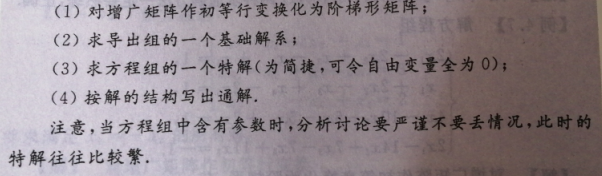六、二次型的基本内容和重要结论
$\color{red}\textbf{二次型的定义}$

二次型是n个变量上的二次齐次多项式。下面给出一个、两个、和三个变量的二次形式：（注意齐次这个定义很重要，每一项都是二次的，而不是二次函数可以有一次项，可以有常数项。）将上面的多项式写成矩阵的形式：
$f(x_1,x_2,...,x_i)=\mathbf{x^TAx}$
其中$\mathbf{A}=[a_{ij}]$且$\mathbf{A}^T=\mathbf{A}$是一个对称矩阵，那么称$A$是二次型的矩阵，秩$r(A)$称为二次型的秩，记为$r(f)$

$\color{red}\textbf{标准形，规范形，正定二次型}$

标准形：如果二次型中只含有变量的平方项。
规范形：在标准形中，各平方项的系数为1，-1，0。
正负惯性指数:在二次型$x^TAx$的标准形中，正平方项的个数称为二次型的正惯性指数，负平方项的系数称为二次型的负惯性指数。
正定二次型：对二次型$x^TAx$，如果对任何$x\neq0$，恒友$x^TAx>0$，则称二次型是正定二次型，且实对称矩阵$A$是正定矩阵。


展开全文机器学习 线性代数
• 线性代数基础知识整理行列式上（三角行列式副对角线行列式特殊拉普拉斯展开式范德蒙德行列式行和列和相等的行列式分块矩阵的行列式行列式公式矩阵矩阵和伴随矩阵秩的关系特征值和特征向量施密特正交化二次型正定...
线性代数基础知识整理行列式上（下）三角行列式副对角线行列式特殊拉普拉斯展开式范德蒙德行列式行和列和相等的行列式分块矩阵的行列式行列式公式矩阵矩阵和伴随矩阵秩的关系特征值和特征向量施密特正交化二次型正定矩阵
行列式
上（下）三角行列式
$\left|\begin{array}{cccc}a_{11} & 0 & \cdots & 0 \\ 0 & a_{22} & \cdots & 0 \\ \vdots & \vdots & & \vdots \\ 0 & 0 & \cdots & a_{n n}\end{array}\right|=\prod_{i=1}^{n} a_{i i}$
副对角线行列式
$\left|\begin{array}{cccc}0 & \cdots & 0 & a_{1, n} \\ 0 & \cdots & a_{2, n-1} & 0 \\ \vdots & & \vdots & \vdots \\ a_{n 1} & \cdots & 0 & 0\end{array}\right|$
$=(-1)^{\frac{n(n-1)}{2}} a_{1 n} a_{2, n-1} \ldots a_{n}$
特殊拉普拉斯展开式
$\left|\begin{array}{cc}A_{m \times m} & O \\ O & B_{n \times n}\end{array}\right|=\left|\begin{array}{cc}A & C \\ O & B\end{array}\right|$
$=\left|\begin{array}{ll}A & O \\ C & B\end{array}\right|=|A||B|$
范德蒙德行列式
$\left|\begin{array}{cc}O & A_{m \times m} \\ B_{n \times n} & O\end{array}\right|=\left|\begin{array}{cc}C & A \\ B & O\end{array}\right|$
$=\left|\begin{array}{cc}O & A \\ B & C\end{array}\right|=(-1)^{m n}|A||B|$
$\left|\begin{array}{cccc}1 & 1 & \cdots & 1 \\ x_{1} & x_{2} & \cdots & x_{n} \\ x_{1}^{2} & x_{2}^{2} & \cdots & x_{n}^{2} \\ \vdots & \vdots & & \vdots \\ x_{1}^{n-1} & x_{2}^{n-1} & \cdots & x_{n}^{n-1}\end{array}\right|$
$=\prod_{1 \leqslant i
行和列和相等的行列式
$\left|\begin{array}{ccccc}a & b & b & \cdots & b \\ b & a & b & \cdots & b \\ b & b & a & \cdots & b \\ \vdots & \vdots & \vdots & & \vdots \\ b & b & b & \cdots & a\end{array}\right|_{n \times n}$
$=[a+(n-1) b](a-b)^{n-1}$
分块矩阵的行列式
$\left|\begin{array}{cc}A & B \\ 0 & D\end{array}\right|=|A||D|$
$\left|\begin{array}{ll}A & B \\ C & D\end{array}\right|$
$\stackrel{\operatorname{exist} A^{-1}}{=}|A|\left|A-C A^{-1} B\right|$
$\stackrel{\operatorname{exist} D^{-1}}{=}|D|\left|A-B D^{-1} C\right|$
行列式公式
$|k A|=k^{n}|A|$
$\left|A^{*}\right|=|A|^{n-1}$
矩阵
$A+B=B+A$
$(A+B)+C=A+(B+C)$
$k(A+B)=k A+k B$
$(k+l) A=k A+l A$
$(A B) C=A(B C)$
$A(B+C)=A B+A C$
$(A+B) C=A C+B C$
$(k A) B=A(k B)=k(A B)$
$\left[\begin{array}{ll}A_{1} & A_{2} \\ A_{3} & A_{4}\end{array}\right]+\left[\begin{array}{ll}B_{1} & B_{2} \\ B_{3} & B_{4}\end{array}\right]$
$=\left[\begin{array}{ll}A_{1}+B_{1} & A_{2}+B_{2} \\ A_{3}+B_{3} & A_{4}+B_{4}\end{array}\right]$
$k\left[\begin{array}{ll}A & B \\ C & D\end{array}\right]=\left[\begin{array}{ll}k A & k B \\ k C & k D\end{array}\right]$
$\left[\begin{array}{ll}A & B \\ C & D\end{array}\right]\left[\begin{array}{ll}X & Y \\ Z & W\end{array}\right]$
$=\left[\begin{array}{ll}A X+B Z & A Y+B W \\ C X+D Z & C Y+D W\end{array}\right]$
$\left[\begin{array}{ll}A & O \\ O & B\end{array}\right]^{n}=\left[\begin{array}{ll}A^{n} & O \\ O & B^{n}\end{array}\right]$
$A^{-1}=\frac{1}{|A|} A^{*}$
$\left(A^{-1}\right)^{-1}=A$
$(k A)^{-1}=\frac{1}{k} A^{-1}(k \neq 0)$
$(A B)^{-1}=B^{-1} A^{-1}$
$(A B)^{T}=B^{T} A^{T}$
$\left(A^{T}\right)^{-1}=\left(A^{-1}\right)^{T}$
$A A^{*}=A^{*} A=|A| E$
$A^{*}=|A| A^{-1}$
$A=|A|\left(A^{*}\right)^{-1}$
$\left(A^{T}\right)^{*}=\left(A^{*}\right)^{T}$
$\left(A^{-1}\right)^{*}=\left(A^{*}\right)^{-1}$
$(A B)^{*}=B^{*} A^{*}$
$\left(A^{*}\right)^{*}=|A|^{n-2} A$
$(k A)^{*}=k^{n-1} A$
$A^{-1}+B^{-1}$
$=A^{-1}\left(E+A B^{-1}\right)$
$=A^{-1}(B+A) B^{-1}$
矩阵和伴随矩阵秩的关系
\begin{aligned} &r\left(A^{*}\right)=\left\{\begin{array}{ll} n & r(A)=n \\ 1 & r(A)=n-1 \\ 0 & r(A) \leqslant n-2 \end{array}\right. \end{aligned}
特征值和特征向量

矩阵
特征值
特征向量

$A$
$\lambda$
$\xi$

$kA$
$k\lambda$
$\xi$

$A^{k}$
$\lambda^{k}$
$\xi$

$f(A)$
$f(\lambda)$
$\xi$

$A^{-1}$
$\lambda^{-1}$
$\xi$

$A^{*}$
$\frac{\operatorname{det}(A)}{\lambda}$
$\xi$

$P^{-1} A P$
$\lambda$
$P^{-1} \xi$

施密特正交化
$\beta_{1}=\alpha_{1}$
$\beta_{2}=\alpha_{2}-\frac{\left(\alpha_{2}, \beta_{1}\right)}{\left(\beta_{1}, \beta_{1}\right)} \beta_{1}$
$\beta_{3}=\alpha_{3}-\frac{\left(\alpha_{3}, \beta_{1}\right)}{\left(\beta_{1}, \beta_{1}\right)} \beta_{1}-\frac{\left(\alpha_{3}, \beta_{2}\right)}{\left(\beta_{2}, \beta_{2}\right)} \beta_{2}$
二次型
合同矩阵：当且仅当存在一个可逆矩阵 $C$ 使得 $C^{T} A C=B,$ 则矩阵 $A$ 合同于矩阵 $C,$ 记作 $A \simeq B$;
合同关系 是一个等价关系, 具有:

自反性
对称性
传递性
合同矩阵的秩相同
矩阵 $A, B$ 合同等价于 $A, B$ 具有相同的正、负惯性指数, 即正、负特征值的个数相等;

正定矩阵
正定矩阵：若对任意非零向量 $x$ 有 $x^{T} A x>0$ 恒成立, 则矩阵 $A$ 是一个正定矩阵。
正定矩阵的性质:

行列式恒为正
必须是对称矩阵
主对角线元素必须为正
必须是可逆矩阵
与单位矩阵合同
逆矩阵也是正定矩阵
两个正定矩阵的和也是正定矩阵
正数与正定矩阵的乘积也是正定矩阵
顺序主子式均为正
存在实可逆矩阵C，使A=C′C
存在秩为n的m×n实矩阵B，使A=B′B
存在主对角线元素全为正的实三角矩阵R，使A=R′R

正定矩阵的充要条件：
(1)n 元实二次型 $f\left(x_{1}, \ldots, x_{n}\right)$ 正定 $\Leftrightarrow$ 它的正惯性指数为 $\mathrm{n} ;$
(2) 一个实对称矩阵 A 正定 $\Leftrightarrow \mathrm{A}$ 与 $\mathrm{E}$ 合同，即?可逆矩阵 $\mathrm{C},$ 使得 $A=C^{T} C$;
(3) 实二次型 $f\left(x_{1}, \ldots, x_{n}\right)=\sum_{i=1}^{n} \sum_{j=1}^{n} a_{i j} x_{i} x_{j}=X^{T} A X$ 是正定的 $\Leftrightarrow$ A的顺序主子式全大于零;
(4) 一个实对称矩阵 A 正定 $\Leftrightarrow$ A 的特征值全大于零;
(5) 一个实对称矩阵 A 正定 $\Leftrightarrow \mathrm{A}$ 的顺序主子式全大于零;
(6)A, $\mathrm{B}$ 是实对称矩阵，则 $C=\left(\begin{array}{cc}A & 0 \\ 0 & B\end{array}\right)$ 正定 $\Leftrightarrow \mathrm{A}, mathrm{B}$均正定；
(7)A 实对称矩阵，A 正定 $\Leftrightarrow \exists$ 正定矩阵 B，使得 $A=B^{k}, \quad(\mathrm{k}$ 为任意正效数 $)$ 。
正定矩阵的判定的方法:
根据正定矩阵的定义及性质，判别对称矩阵A的正定性有两种方法：
（1）求出A的所有特征值。若A的特征值均为正数，则A是正定的；若A的特征值均为负数，则A为负定的。
（2）计算A的各阶主子式。若A的各阶主子式均大于零，则A是正定的；若A的各阶主子式中，奇数阶主子式为负，偶数阶为正，则A为负定的。


展开全文线性代数
• ## R语言矩阵运算

千次阅读 2012-06-20 15:07:38
主要包括以下内容： 创建矩阵向量；...行列式的值；特征值与特征向量；QR分解；奇异值分解；广义逆；backsolve与fowardsolve函数；取矩阵的上下三角元素；向量化算子等. 1 创建一语言 matrix function c class
• 其中部分习题摘自《660》一、普通行列式（1） （2）上/下三角行列式 （3）范德蒙行列式 例： 这道题需要注意的是，由于公式（1），导致范德蒙德除了定义中的情况，在某些情况下还可以用于其转置矩阵。（4）...
• ③、上、下三角行列式（ ）：主对角元素的乘积； ④、 和 ：副对角元素的乘积 ； ⑤、拉普拉斯展开式： 、 ⑥、范德蒙行列式：大指标减小指标的连乘积； ⑦、特征值； 6. 对于 阶行列式 ，恒有： ，其中 为 阶主子...考研数学
• ③、上、下三角行列式（ ）：主对角元素的乘积； ④、 和 ：副对角元素的乘积 ； ⑤、拉普拉斯展开式： 、 ⑥、范德蒙行列式：大指标减小指标的连乘积； ⑦、特征值； 6. 对于 阶行列式 ，恒有： ，其中 为 阶主子...
• 下三角矩阵：tril 矩阵的转置 若A为实矩阵，则两种转置结果相同 矩阵的旋转 矩阵的翻转 矩阵的求逆 矩阵求 行列式 矩阵的秩 矩阵的迹 矩阵和向量的范数 矩阵的条件数 矩阵的...matlab
• det 矩阵的行列式值 eig 矩阵的特征值 inv 矩阵的逆 norm 矩阵的范数值 normest 矩阵的2-范数值 rank 矩阵的秩 orth 矩阵的正交化运算 rcond 矩阵的逆条件数值 trace 矩阵的迹 triu 上三角变换 tril ...matlab 矩阵运算
• 行列式的值；特征值与特征向量；QR分解；奇异值分解；广义逆；backsolve与fowardsolve函数；取矩阵的上下三角元素；向量化算子等。 1、创建向量 > x=c(1,2,3,4) > x  1 2 3 4 2、创建矩阵 在R中可以用...
• 行列式的值；特征值与特征向量；QR分解；奇异值分解；广义逆；backsolve与fowardsolve函数；取矩阵的上下三角元素；向量化算子等.1 创建一个向量在R中可以用函数c()来创建一个向量，例如：> x=c(1,2,3,4)> x[1...e指数上矩阵运算
• 行列式的值；特征值与特征向量；QR分解；奇异值分解；广义逆；backsolve与fowardsolve函数；取矩阵的上下三角元素；向量化算子等.1 创建一个向量在R中可以用函数c()来创建一个向量，例如：> x=c(1,2,3,4)> x[1...
• 行列式的值；特征值与特征向量；QR分解；奇异值分解；广义逆；backsolve与fowardsolve函数；取矩阵的上下三角元素；向量化算子等. 1 创建一个向量 在R中可以用函数c()来创建一个向量，例如： > x=c(1,2,3,4) ...
• 矩阵的行列式计算 矩阵的幂运算矩阵的幂运算 矩阵的特征值运算 求矩阵行数/列数/维数 矩阵的简单函数 一些特殊矩阵的生成 矩阵元素的定位和获取 矩阵的对角抽取 上三角矩阵和下三角矩阵抽取 向量的生成 1....MATLAB
• 前言：国内本科的线性代数课程以及考试实际是在玩一些特殊且固定的套路，对于更一般的矩阵乘法，高斯消元，...特殊的矩阵应用（包括乘法，求逆，行列式特征值） 1. 对角矩阵 2. 上下三角矩阵 3. 三对角矩阵 ...
• 函数概览函数调用方式解释det(A)计算A的行列式diag(A)取A对角元构成向量或利用向量A生成对角阵expm(A)计算A的矩阵指数运算inv(A)计算A的逆矩阵rank(A)计算A的秩tril(A)将A化为下三角矩阵（其余元素变为0）[V D]=eig...
• 1、行列式 2、代数余子式 3、伴随矩阵 4、逆矩阵 4.1概念 4.2求解 5、矩阵运算 6、矩阵转置 7、特殊矩阵 （1）零矩阵，所有元素都等于零，也可以记为0 （2）单位矩阵，对角线上都为1，其他...
• 5.6.3求4阶矩阵的特征值和特征向量 5.7小结 第6章解多元一次线性方程组 6.1多元一次方程组简介 6.2解实系数多元一次方程组 6.2.1解实系数二元一次方程组 6.2.2解实系数三元一次方程组 6.2.3解实系数四元一次...
• 矩阵运算1 1.1 实矩阵相乘1 1.2 复矩阵相乘4 1.3 一般实矩阵求逆8 1.4 一般复...行列式33 1.10 矩阵的三角分解36 1.11 一般实矩阵的QR分解41 1.12 一般实矩阵的奇异分解46 1.13 求广义逆的奇异分解法61 第2章...
• 2.4.2．上三角阵和下三角阵的抽取 19 2.4.3．矩阵的变维 20 2.4.4．矩阵的变向 20 2.4.5．矩阵的比较关系 22 2.4.6．矩阵元素的数据变换 22 2.4.7．矩阵逻辑运算 23 2.5. 矩阵分解 24 2.5.1 Cholesky分解 24 2.5.2 ...
• ## C++常用算法程序集

热门讨论 2011-03-24 09:15:32
1.7 求一般行列式25 1.8 求矩阵的秩29 1.9 对称正定矩阵的乔里斯基分解与行列式33 1.10 矩阵的三角分解36 1.11 一般实矩阵的QR分解41 1.12 一般实矩阵的奇异分解46 1.13 求广义逆的奇异分解法61 第2章 ...
• 1.9 对称正定矩阵的乔里斯基分解与行列式求值33 1.10 矩阵的三角分解36 1.11 一般实矩阵的QR分解41 1.12 一般实矩阵的奇异值分解46 1.13 求广义逆的奇异值分解法61 第2章 矩阵特征值与特征向量的计算75 2.1 求对称三...
• 4.7 求一般行列式的值 4.8 求矩阵的值 4.9 对称正定矩阵的乔里斯基分解与列式求值 4.10 矩阵的三角分解 4.11 一般实矩阵的QR分解 4.12 一般实矩阵的奇异值分解 4.13 求广义逆的奇异值分解法 第5章 矩阵特征值与特征...